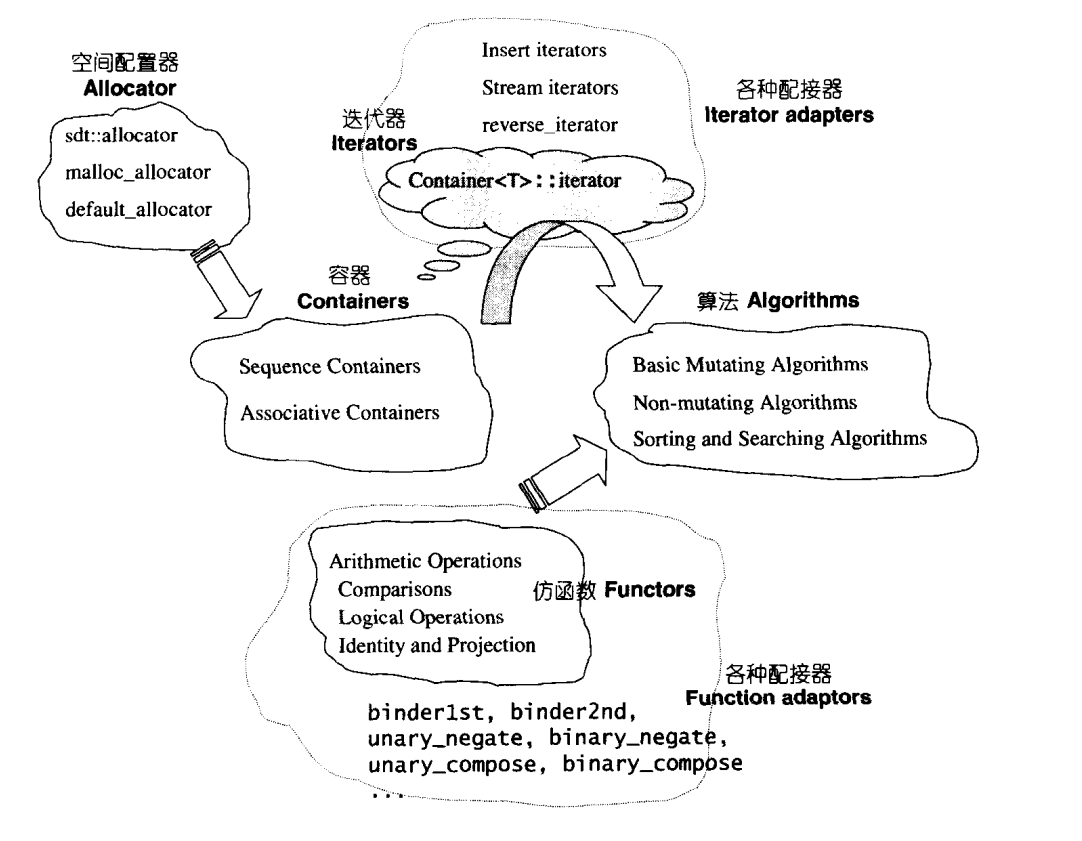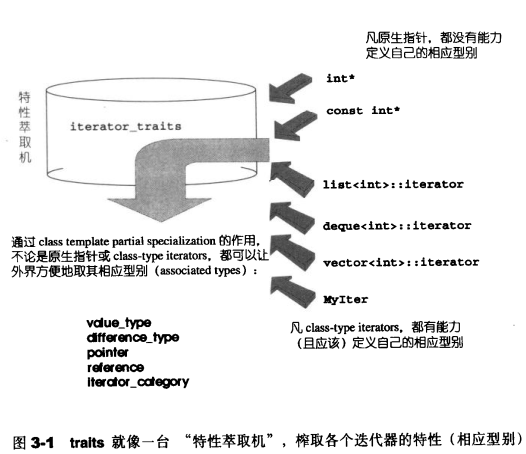# C++ STL 源码剖析之 Traits 编程技法¶

## 0.导语¶``````template<class _Tp>
struct iterator_traits<_Tp*>
{
typedef ptrdiff_t difference_type;
typedef typename _Tp::value_type value_type;
typedef typename _Tp::pointer pointer;
typedef typename _Tp::reference reference;
typedef typename _Tp::iterator_category iterator_category;
};
``````

## 1.template 参数推导¶

``````template <class I>
inline
void func(I iter) {
func_impl(iter, *iter); // 传入iter和iter所指的值，class自动推导
}
``````

``````template <class I, class T>
void func_impl(I iter, T t) {
T tmp; // 这里就是迭代器所指物的类别
// ... 功能实现
}

int main() {
int i;
func(&i);
}
``````

## 2.声明内嵌型别¶

``````template <class I, class T>
T func_impl(I iter, T t) {
T tmp; // 这里就是迭代器所指物的类别
// ... 功能实现
}
template <class T>
(*T) func(T t) { // !!!Wrong code
return func_impl(t, *t); // forward the task to func_impl
}
int main() {
int i  =10;
cout<<func(&i)<<endl; // !!! Can’t pass compile
}
``````

``````template <class T>
struct MyIter {
typedef T value_type; // 内嵌型别声明
T* ptr;
MyIter(T* p = 0) : ptr(p) {}
T& operator*() const { return *ptr; }
};

template <class I>
typename I::value_type
func(I ite) {
std::cout << "class version" << std::endl;
return *ite;
}
int main() {
// ...
MyIter<int> ite(new int(8));
cout << func(ite);  // 输出8
}
``````

``````int *p = new int(5);
cout<<func(p)<<endl; // error
``````

## 3.救世主 Traits¶

（1）函数偏特化

``````template <class T>
struct MyIter {
typedef T value_type; // 内嵌型别声明
T* ptr;
MyIter(T* p = 0) : ptr(p) {}
T& operator*() const { return *ptr; }
};

template <class I>
typename I::value_type
func(I ite) {
std::cout << "class version" << std::endl;
return *ite;
}
template <class I>
I
func(I* ite) {
std::cout << "pointer version" << std::endl;
return *ite;
}
template <class I>
I func(const I* ite) {
std::cout << "const pointer version" << std::endl;
return *ite;
}
int main() {
// ...
MyIter<int> ite(new int(8));
cout << func(ite)<<endl;
int *p = new int(52);
cout<<func(p)<<endl;
const int k = 3;
cout<<func(&k)<<endl;
}
``````

``````class version
8
pointer version
52
const pointer version
3
``````

（2）加入中间层``````#include <iostream>

template <class T>
struct MyIter {
typedef T value_type; // 内嵌型别声明
T* ptr;
MyIter(T* p = 0) : ptr(p) {}
T& operator*() const { return *ptr; }
};
// class type
template <class T>
struct iterator_traits {
typedef typename T::value_type value_type;
};
// 偏特化1
template <class T>
struct iterator_traits<T*> {
typedef T value_type;
};
// 偏特化2
template <class T>
struct iterator_traits<const T*> {
typedef T value_type;
};

template <class I>
typename iterator_traits<I>::value_type
// 首先询问iterator_traits<I>::value_type,如果传递的I为指针,则进入特化版本,iterator_traits直接回答;如果传递进来的I为class type,就去询问T::value_type.
func(I ite) {
std::cout << "normal version" << std::endl;
return *ite;
}
int main() {
// ...
MyIter<int> ite(new int(8));
std::cout << func(ite)<<std::endl;
int *p = new int(52);
std::cout<<func(p)<<std::endl;
const int k = 3;
std::cout<<func(&k)<<std::endl;
}
``````

https://juejin.im/post/5b1a43fb51882513bf1795c6

https://www.cnblogs.com/mangoyuan/p/6446046.html

http://www.cppblog.com/nacci/archive/2005/11/03/911.aspx